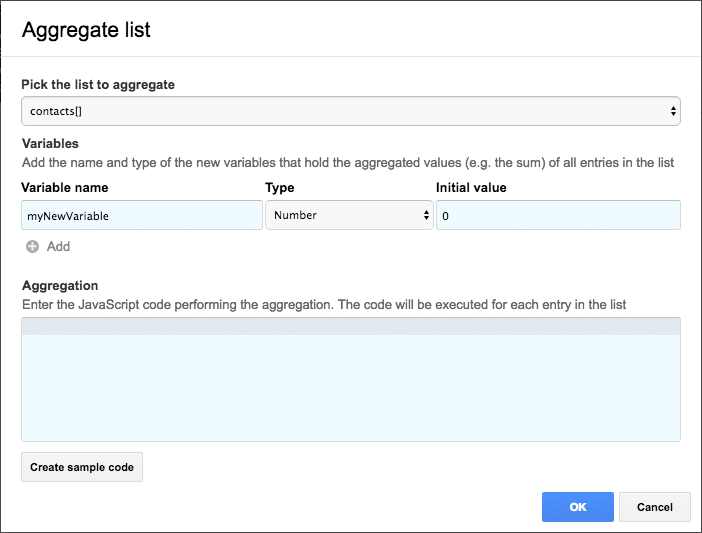# Aggregate

 Perform aggregations on all entries in the list 1
 list[].attribute1
 list[].attribute1
 list[].attribute2
 list[].attribute2
 list[].attribute3
 list[].attribute3

The `Aggregate` building block allows you to sum up values in your table or to perform other calculations that require iterating through all the rows of your table

### Use cases

• Sum up values of a given sheet and include them into status reports

### Configuration

Click on the bold text in the title of the building block to open the configuration dialog.After adding the script to your flow, click on the keyboard icon in the input parameter section.

If you are summing up data of a particular column in your table to calculate the total, you will end up with a new variable holding the calculated value.

#### Initialize new variables

First of all you'll have to initialize the new variables that you want to create. Use the result prefix when initializing your variables. If you want to create two variables called `subtotal` and `vat` , you'll have to initialize them like this:

``result.vat = 0; result.subtotal = 0;``

You may use Ultradox variables in your statements, they will be evaluated before the script gets executed. So in case you want to initialize the `subtotal` variable with an existing value you can use an expression like this:

``result.vat = 0; result.subtotal = \${startvalue}``

#### Do the math

After initializing the new variables, you can use them in the expression that will be calculated for each row in your data:

``result.vat += row.amount * row.quantity * \${vatrate} / 100; result.subtotal += row.amount * row.quantity``